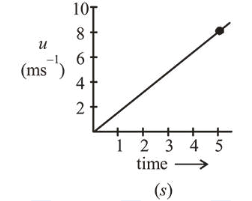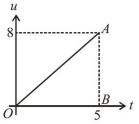# The speed verses time graph for a particle is shown in the figure.Question:

The speed verses time graph for a particle is shown in the figure. The distance travelled (in $\mathrm{m}$ ) by the particle during the time interval $t=0$ to $t=5 \mathrm{~s}$ will be_____________Solution:

(20)Distance travelled = Area of speed-time graph

$=\frac{1}{2} \times 5 \times 8=20 \mathrm{~m}$